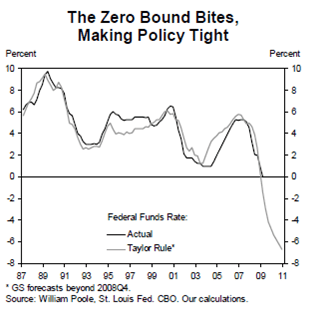Posted by: Dirk | January 19, 2009

## The end of inflation-targeting (simplistic)

Paul Krugman does some zero lower bound blogging. The main graph below is introduced by this:

Today, however, with expected inflation roughly zero and a recession that is the fruit of past irrational exuberance, conventional monetary policy has run out of room. That’s the point of the Goldman Sachs exercise, shown below, which asks what the familiar Taylor rule would prescribe for monetary policy over the next few years; the answer is a Fed funds rate of -6, which isn’t possible.For those with some formal knowledge of the IS/LM model I have put together a sketch which should reveal the logic behind the zero bound problem. (This interpretation of reality is a bit simplistic, but it makes the main point more explicit. If you don’t like the connection, regard the IS and LM curves for 2007/08 only and take the others as examples for equilibria with non-negative interest rates.)

Below, I have sketched out the IS/LM model. I assume that the economy is hit by a demand shock every once in a while. Thus, the IS curve shifts leftwards. This leads to deflationary tendencies, triggering a monetary response (given by the Taylor rule). The money supply increases in order to stabilize inflation. This has been performed in 1991, 2000 and 2007/08. (Compare with graph above.)The problem is that in order to stabilze output around potential output (the vertical line), what is now needed is a negative nominal interest rate. This clearly is not feasable. Nobody would keep money in a bank when the bank would charge negative interest rates. You pay the bank for holding your money. Cash does the trick at no cost, so people would opt for cash.

Advertisements